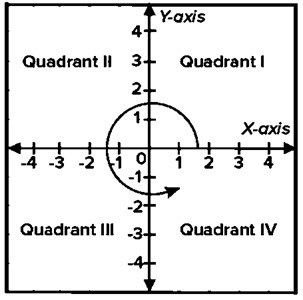Given coordinate points (x, y), we have to determine its quadrant using the Rust program.
Submitted by Nidhi, on October 01, 2021

Problem Solution:

Here, we will read the coordinate points from the user. Then we will determine the quadrant and print the result.

There are four Quadrants based on the origin (Meeting point of the two axes).

• In the first quadrant, both x and y have positive values.
• In the second quadrant, x is negative and y is positive.
• In the third quadrant, x and y are negative
• In the fourth quadrant, x is positive and y is negative.

Consider the following graph,Program/Source Code:

The source code to read coordinate points and determine their quadrant is given below. The given program is compiled and executed successfully.

```// Rust program to read coordinate points

use std::io;

fn main()
{
let mut X:i32   =0;
let mut Y:i32   =0;
let mut res:i32 =0;

let mut input1 = String::new();
let mut input2 = String::new();

println!("Enter value of X: ");
X = input1.trim().parse().expect("Not a valid number");

println!("Enter value of Y: ");
Y = input2.trim().parse().expect("Not a valid number");

if X == 0 && Y == 0
{
println!("Point ({0}, {1}) lies at the origin", X, Y);
}
else if X > 0 && Y > 0
{
println!("Point ({0}, {1}) lies in the First quadrant", X, Y);
}
else if X < 0 && Y > 0
{
println!("Point ({0}, {1}) lies in the Second quadrant", X, Y);
}
else if X < 0 && Y < 0
{
println!("Point ({0}, {1}) lies in the Third quadrant", X, Y);
}
else if X > 0 && Y < 0
{
println!("Point ({0}, {1}) lies in the Fourth quadrant", X, Y);
}
}
```

Output:

```RUN 1:
Enter value of X:
12
Enter value of Y:
15
Point (12, 15) lies in the First quadrant

RUN 2:
Enter value of X:
-5
Enter value of Y:
-10
Point (-5, -10) lies in the Third quadrant
```

Explanation:

Here, we read the value of coordinates from the user. Then we determined the quadrant and printed the result.